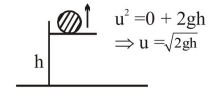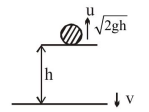# A helicopter reises from rest on the ground vertically upwards with a constant acceleration g

Question:

A helicopter reises from rest on the ground vertically upwards with a constant acceleration g. A food packet is dropped from the helicopter when it is a height $h$. The time taken by the packet to reach the ground is close to $[g$ is the acceleration due to gravity] :

1. $\mathrm{t}=\sqrt{\frac{2 \mathrm{~h}}{3 \mathrm{~g}}}$

2. $\mathrm{t}=1.8 \sqrt{\frac{\mathrm{h}}{\mathrm{g}}}$

3. $\mathrm{t}=3.4 \sqrt{\left(\frac{\mathrm{h}}{\mathrm{g}}\right)}$

4. $\mathrm{t}=\frac{2}{3} \sqrt{\left(\frac{\mathrm{h}}{\mathrm{g}}\right)}$

Correct Option: , 3

Solution:$v^{2}=u^{2}+2 a s$

$v^{2}=2 g h+2 g h$

$v=\sqrt{4 g h}$

$\Rightarrow \sqrt{4 \mathrm{gh}}=\sqrt{2 \mathrm{gh}}+\mathrm{gt}$

$\Rightarrow \mathrm{t}=\sqrt{\frac{4 \mathrm{~h}}{\mathrm{~g}}}-\sqrt{\frac{2 \mathrm{~h}}{\mathrm{~g}}} \Rightarrow 3.4 \sqrt{\frac{\mathrm{h}}{\mathrm{g}}}$